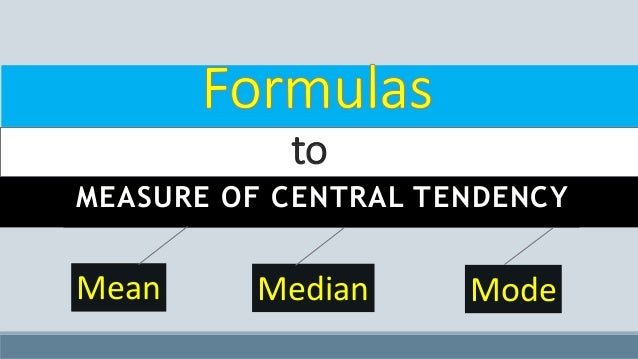Successfully reported this slideshow.Upcoming SlideShare
×

# Formulas to measure_central_tendency_(mean,_median,_mode)

126 views

Published on

These slides are prepared for the students who study statistics ..
Under this formula to central tendency is given:

Published in: Data & Analytics
• Full Name
Comment goes here.

Are you sure you want to Yes No• Be the first to comment

### Formulas to measure_central_tendency_(mean,_median,_mode)

1. 1. MEASURE OF CENTRAL TENDENCY Mean Median Mode to
2. 2. One of the most important objectives of a statistical analysis is to get one single value which represent the entire mass of data that unique value is called an average value. The average value lies between the smallest value & the largest value that central value is also known as measure of central tendency. Measure of Central Tendency
3. 3. Types of Measure of Central Tendency 1. Mean (X) 2. Median (Md) 3. Mode (Mo)
4. 4. 1. Arithmetic Mean The Sum of all observations divided by total number of observations is called Arithmetic mean. It is denoted by X & defined as,
5. 5. Individual series Direct Method Assumed Mean Method Where, n=total number of observations X= Variable value A= Assumed Mean d= X - A 1. Arithmetic Mean
6. 6. Discrete series Direct Method Assumed Mean Method Where, n= 𝛴f X= Variable value A= Assumed Mean d= X - A 1. Arithmetic Mean
7. 7. Continuous series Direct Method: Assumed Mean Method: Step-deviation Method: Where, h= class interval 1. Arithmetic Mean
8. 8. 2. Median If the series is arranging in ascending or descending order of its magnitude then the value of middle term is called as Median. It is divided the entire series into equal parts & lies 50% of the distribution so it is known as positional average. Median is appropriate to calculate the measure of central tendency or average in case of open-end class. And it is also used to test the qualitative data such as intelligence, honesty, beauty etc. It is denoted by Md & defined as:
9. 9. Individual series 2. Median Where, n= total number of observations for individual series.
10. 10. Discrete series 2. Median Where, n= total number of observations for individual series. n= 𝛴f for discrete series
11. 11. Continuous series 2. Median If the series to find the median first of all we identify median class by using formula, Finally, Where, n= 𝛴f f= frequency h= height of the class l= lower limit c.f.= cumulative frequency
12. 12. 3. Mode The most repeated value of a group is called mode or the variate value having the maximum frequency is called mode. It is used to calculate the most repeated value, most common size, most common wage etc. It is unrigidly define average& It is denoted by Mo & defined as:
13. 13. Individual series 3. Mode If we have unrepeated items in such case to find the mode by using the following relation:
14. 14. Continuous series 3. Mode Where, l = lower limit of the model class f1 = Frequency of the model class f0 = Frequency of the pre model class f2= Frequency of the post model class h= height of the class
15. 15. 3. Mode Grouping Method In abnormal problem to find the mode by using the grouping method. In grouping method the following steps are used: Step 1: Prepare the grouping Table. Step 2: Prepare the analysis table. Step 3: Use Formula.
16. 16. For Grouping Method Column I : Frequency itself. Column II : Sum of two frequencies. Column III : Leaving first & sum of two frequencies. Column IV : Sum of three frequencies. Column V : Leaving first & sum of three frequencies. Column VI : Leaving first & second & sum of three frequencies. 3. Mode
17. 17. For e.g. Class 10-20 20-30 30-40 40-50 50-60 60-70 70-80 80-90 90-100 100-110 f 10 5 15 10 20 22 24 6 2 1 Step 1: Prepare the grouping Table. Class Col. I (f) Col. II Col. III Col. IV Col. V Col. VI 10-20 10 20-30 6 31 30-40 15 31 40-50 10 45 50-60 20 52 60-70 22 66 70-80 24 52 80-90 6 32 90-100 2 9 100-110 1 16 25 42 30 3 21 30 46 8
18. 18. Step 2: Prepare the analysis table. Class Col. I Col. II Col. III Col. IV Col. V Col. VI Total 10-20 0 20-30 0 30-40 0 40-50 1 50-60 2 60-70 5 70-80 4 80-90 1 90-100 0 100-110 0 Since , the maximum contribution of the frequency is 5 & which correspond to the model class 60-70. Therefore , M0del Class is (60-70) 3. Mode
19. 19. Step 3: Use Formula If (f1 < f2) then, we use this formula Otherwise, 3. Mode
20. 20. Thank You